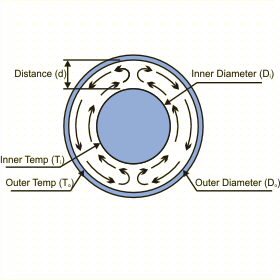Close

This page calculates the natural convection heat transfer between two concentric cylinders maintained at constant temperatures. The calculation is based on Rayleigh number and is valid for Rayleigh numbers below 107The heat transfer between the cylinders (qc) is calculated as:

qc = 2Pi L keff (Ti - To) / ln(Do/Di)

Where L is the length of the cylinders, Ti is the temperature of the inner cylinder and To is the temperature of the outer cylinder, where keff is calculated as:

keff  / k = 0.386(Rac*)1/4(Pr / (0.861+Pr))1/4
where Rac* = Rad (ln(Do/Di))4 / (d3(Di-3/5+Do-3/5)5)
Rad = gB rho2Cp(Ti-To)d3 / kµ

For the above equations Rad is the Rayleigh number based on the distance Ro-Ri, i.e. the difference between the radius of the outer cylinder and the inner cylinder, g the gravitational acceleration, B the coefficient of thermal expansion, k the thermal conductivity, Pr the Prandtl number, a the thermal diffusivity and µ the dynamic viscosity. The equation for keff is valid between 102 < Rac* < 107. For values below 102, keff = k.

In addition, you must define the fluid properties at the film temperature Tf defined as follows, except for the density which is defined at the reference temperature of 20 C.

Tf = (Tp + Ta) / 2

The fluid density at the film temperature is automatically calculated from the following relationship based on the perfect gas law:

Rho = Rhoref (Tref +273) / (Tf + 273)

References

Raithby, G. D., and K. G. T. Hollands, A General Method of Obtaining Approximate Solutions to Laminar and Turbulent Free Convection Problems, in T. F. Irvine and J. P. Hartnett, Eds., Advances in Heat Transfer, Vol. 11, Academic Press, New York, pp 265-315, 1975.

Incropera, De Witt., Fundamentals of Heat and Mass Transfer, 3rd ed., John Wiley & Sons, p563, eq 9.58-9.60, 1990.